Next: The RLS filter Up: number9 Previous: The Kalman filter

An important general form for adaptive filters is the Least Mean Squares (LMS) filter. This type of adaptive filter is easy to implement and is widely used because of this. The filter uses a gradient search technique in order to determine how to improved the filter coefficients. This gradient search is also reason for the basic weakness of the filter: it has a relatively slow convergence rate.

Suppose we consider the N-th order FIR filter,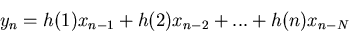(6)

the filter error is,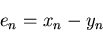(7)

With the LMS filter, we adjust the values of the coefficients,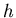, proportional to the error,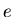,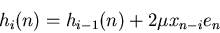(8)

where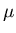is a learning factor'' which controls how strongly the error is weighted. This equation is the consequence of the requirement to minimize the mean square value of, hence the name of the filter.Next: The RLS filter Up: number9 Previous: The Kalman filter
Skip Carter 2008-08-20